# Delete rows and columns of NumPy ndarray

• Last Updated : 21 Apr, 2021

In this article, we will discuss how to delete the specified rows and columns in an n-dimensional array. We are going to delete the rows and columns using numpy.delete() method.

Syntax: numpy.delete(array_name, obj, axis=None)

Let’s discuss with the help of some examples:

Example 1:

Program to create a 2-dimensional array (3 rows and 4 columns) with NumPy and delete the specified row.

## Python3

 `# importing numpy module``import` `numpy as np`` ` `# create an array with integers``# with 3 rows and 4 columns``a ``=` `np.array([[``1``, ``2``, ``3``, ``4``],``              ``[``5``, ``6``, ``7``, ``8``], ``              ``[``9``, ``10``, ``11``, ``12``]])``print``(a)`` ` `# delete 0 th row``data ``=` `np.delete(a, ``0``, ``0``)``print``(``"data  after 0 th row deleted :"``, data)`` ` `# delete 1 st row``data ``=` `np.delete(a, ``1``, ``0``)``print``(``"data  after 1 st  row deleted :"``, data)`` ` `# delete 2 nd row``data ``=` `np.delete(a, ``2``, ``0``)``print``(``"data  after 2 nd  row deleted :"``, data)`

Output: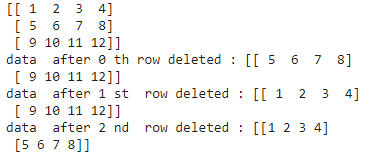Example 2:

Program to create a 2-dimensional array (6 rows and 2 columns) with NumPy and delete the specified columns.

## Python3

 `# importing numpy module``import` `numpy as np`` ` `# create an array with integers with``# 6 rows and 2 columns``a ``=` `np.array([[``1``, ``2``], [``5``, ``6``], [``9``, ``10``, ],``              ``[``78``, ``90``], [``4``, ``89``], [``56``, ``43``]])``print``(a)`` ` `# delete 0 th column``data ``=` `np.delete(a, ``0``, ``1``)``print``(``"data  after 0 th  column  deleted :"``, data)`` ` `# delete 1 st column``data ``=` `np.delete(a, ``1``, ``1``)``print``(``"data  after 1 st  column  deleted :"``, data)`

Output: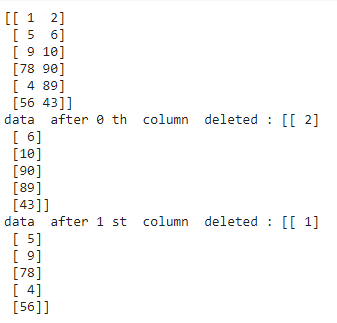Example 3:

Delete both 1 row and 1 column.

## Python3

 `# importing numpy module``import` `numpy as np`` ` `# create an array with integers``# with 3 rows and 3 columns``a ``=` `np.array([[``67``, ``65``, ``45``], ``              ``[``45``, ``67``, ``43``], ``              ``[``3``, ``4``, ``5``]])``print``(``"Original\n"``, a)`` ` `# delete 1 st row``data ``=` `np.delete(a, ``0``, ``0``)``print``(``"data  after 1 st row   deleted :\n"``, data)`` ` `# delete 1 st column``data ``=` `np.delete(a, ``0``, ``1``)``print``(``"data  after 1 st  column  deleted :\n"``, data)`

Output: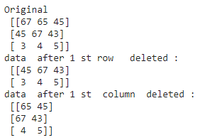Example 4:

We can delete n number of rows at a time by passing row numbers as a list in the obj argument.

Syntax: numpy.delete(array_name, [row1,row2,.row n], axis=None)

## Python3

 `# importing numpy module``import` `numpy as np`` ` `# create an array with integers ``# with 3 rows and 3 columns``a ``=` `np.array([[``67``, ``65``, ``45``], ``              ``[``45``, ``67``, ``43``], ``              ``[``3``, ``4``, ``5``]])``print``(``"Original\n"``, a)`` ` `# delete 1 st row and 2 nd ``# row at a time``data ``=` `np.delete(a, [``0``, ``1``], ``0``)``print``(``"data  after 1 st  and 2 ns row deleted :\n"``, data)`

Output: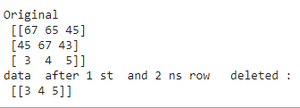Example 5:

We can delete n number of columns at a time by passing column numbers as a list in the obj argument.

Syntax: numpy.delete(array_name, [column number1,column number2,.column number n], axis=None)

## Python3

 `# importing numpy module``import` `numpy as np`` ` `# create an array with integers ``# with 3 rows and 3 columns``a ``=` `np.array([[``67``, ``65``, ``45``],``              ``[``45``, ``67``, ``43``], ``              ``[``3``, ``4``, ``5``]])``print``(``"Original\n"``, a)`` ` ` ` `# delete 1 st column and 3 rd ``# column at a time``data ``=` `np.delete(a, [``0``, ``2``], ``1``)``print``(``"data  after 1 st and 3 rd column  deleted :\n"``, data)`

Output: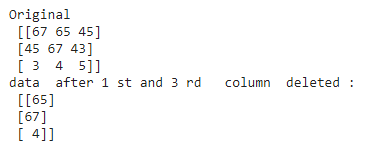My Personal Notes arrow_drop_up